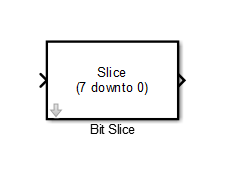# Bit Slice

Return field of consecutive bits from input signal

• Library:
• HDL Coder / Logic and Bit Operations

•## Description

The Bit Slice block returns a field of consecutive bits from the input signal. Specify the lower and upper boundaries of the bit field by using zero-based indices in the LSB Position and MSB Position parameters. For an input word size `WS`, LSB Position and MSB Position must satisfy these constraints:

`WS > MSB Position >= LSB Position >= 0`

## Ports

### Input

expand all

Input signal on which the bit-slice is performed. The input signal has a maximum bit width of 128.

Data Types: `int8` | `int16` | `int32` | `int64` | `uint8` | `uint16` | `uint32` | `uint64` | `Boolean` | `fixed point`

### Output

expand all

Output signal that is bit-sliced. The word length of the output is computed as `(MSB Position - LSB Position) + 1`, where MSB Position and LSB Position are block parameters.

Data Types: `uint8` | `uint16` | `uint32` | `uint64` | `fixed point`

## Parameters

expand all

Specifies the bit position (zero-based) of the most significant bit (MSB) of the field to extract.

For an input word size `WS`,MSB Position must satisfy these constraints:

`WS > MSB Position >= LSB Position >= 0`

#### Programmatic Use

 Block parameter: `lidk` Type: string scalar | character vector Value: positive integer value such that satisfies the constraints mentioned previously Default: `'7'`

Specifies the bit position (zero-based) of the least significant bit (LSB) of the field to extract.

For an input word size `WS`, LSB Position must satisfy these constraints:

`WS > MSB Position >= LSB Position >= 0`

#### Programmatic Use

 Block parameter: `ridk` Type: string scalar | character vector Value: positive integer value such that satisfies the constraints mentioned previously Default: `'0'`

## Version History

Introduced in R2014a